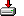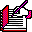#ora2sas.sas```%macro ora2sas (
ORATABLE =
, OUT      =
, OUTYPE   = VIEW
, ORAUSER  =
, ORAPW    =
, INSTANCE =
, SASSEL   = *
, ORASEL   = *
, SASWHERE =
, ORAWHERE =
, ORAORDER =
, SASORDER =
, CFMTON   =
);

%*----------------------------------------------------------------;
%* Author: Richard DeVenezia
%*
%* called from: various
%* ORATABLE =            %* Table in Oracle to retrieve from;
%* OUT      =            %* SAS Data set name to create;
%* OUTYPE   =            %* SAS Data type (VIEW or TABLE);
%* ORAUSER  =            %* Oracle username;
%* INSTANCE =            %* Oracle database instance to connect to;
%* SASSEL   =            %* What columns should be selected in SAS;
%* ORASEL   =            %* What columns should be selected in Oracle;
%* SASWHERE =            %* Where clause to apply in SAS;
%* ORAWHERE =            %* Where clause to apply in Oracle;
%* CFMTON   =            %* Reformat the character columns based on their values in
%*                          this number of firstmost rows;
%*                          Only the SAS format is changed, not the underlying column width
%*
%* mod:
%*  5/10/99 rad add handling of tables with a column of type ROWID
%*              (ROWID columned tables are not translated to SAS by default)
%*              add content based format metricing of character columns
%*----------------------------------------------------------------;

%local ROWIDS;   %* list of oracle columns which are of type ROWID;
%local i;

%if (%superq (ORATABLE) eq ) %then %do;
%put ERROR: Missing Oracle table name, use ORATABLE=;
%goto ByeBye;
%end;

%if (%superq (OUT) eq ) %then %do;
%put ERROR: Missing output data set name, use OUT=;
%goto ByeBye;
%end;

%if (%superq (ORASEL) eq ) %then %do;
%put ERROR: Missing Oracle selection, use ORASEL=;
%goto ByeBye;
%end;

%if (%superq (SASSEL) eq ) %then %do;
%put ERROR: Missing SAS selection, use SASSEL=;
%goto ByeBye;
%end;

%let OUTYPE = %upcase (&OUTYPE);

%if %superq (OUTYPE) ne TABLE and
%superq (OUTYPE) ne VIEW
%then %do;
%put ERROR: OUTYPE= must be TABLE or VIEW;
%goto ByeBye;
%end;

%local OUTLIB OUTMEM;

%let OUTMEM = %scan (&OUT,1,%str(%());

%if (%scan (&OUTMEM,2,.) ne ) %then %do;
%let OUTLIB = %scan (&OUTMEM,1,.);
%let OUTMEM = %scan (&OUTMEM,2,.);
%end;
%else %do;
%let OUTLIB = WORK;
%let OUTMEM = &OUTMEM;
%end;

%local MEMTYPE;

%if &OUTYPE = TABLE %then %let MEMTYPE=DATA;

proc sql;

%if %sysfunc (EXIST (&OUTLIB..&OUTMEM))      %then drop table &OUTLIB..&OUTMEM;;
%if %sysfunc (EXIST (&OUTLIB..&OUTMEM,VIEW)) %then drop view  &OUTLIB..&OUTMEM;;

connect to ORACLE (USER=&ORAUSER ORAPW=&ORAPW PATH="@&INSTANCE");

%* check if the oracle table has columns of ROWID type;

create view oracols as select *
from connection to ORACLE
( select column_name, data_type, column_id
from ALL_TAB_COLUMNS
where table_name=%str(%'%upcase(&ORATABLE)%')
order by column_id
);

reset noprint;
select column_n into :ROWIDS separated by ' ' from oracols where data_typ in ('ROWID');

%if &sqlobs > 0 %then %do;
%* blech - need to explicitly name each column AND ROWIDTOCHAR the rowid column;
%*         also, column names should be enclosed in double quotes in case a column name is
%*         an Oracle restricted word (i.e. a column named mode would have to be specified
%*         as "mode", otherwise Oracle would think the function mode was being indicated;

%if (%superq(ORASEL) = %str(*)) %then %do;
select
case
when data_typ ne 'ROWID' then """" || trim(column_n) || """"
else "ROWIDTOCHAR (""" || trim(column_n) || """) as """ || trim(column_n) || """"
end
into :ORASEL separated by ', '
from oracols
order by column_i;
%end;
%else %do;

%* assume the user knows what they are doing and will not work with ROWID variables ?
%* if they want to work with ROWID variables, the ORASEL should look something like:
%* %STR (ROWIDTOCHAR(ROW_ID), ..., ...) ;

%end;
%end;

create &OUTYPE &OUT as
select &SASSEL
from connection to ORACLE

(select &ORASEL from &ORATABLE
%if (%superq (ORAWHERE) ne ) %then
where &ORAWHERE;
%if (%superq (ORAORDER) ne ) %then
order by &ORAORDER;
)
%if (%superq (SASWHERE) ne ) %then
where &SASWHERE;
%if (%superq (SASORDER) ne ) %then
order by &SASORDER;

;
disconnect from ORACLE;
quit;

%if (&CFMTON > 0) %then %do;
proc contents noprint data=&OUT out=_CNTNTS_;
run;

%local maxlenfs;

%* put SQL select statements in macro var maxlenfs.
%* the statement will determine maximum length of character
%* values in character columns in first CFMTON rows and
%* place that length in a <var> \$<maxlen-of-var> construct;

proc sql noprint;
select
quote(trim(name)) || " || ' \$' || "
|| "trim(left(put(MIN(200, MAX(LENGTH(" || trim (NAME) || "))),best8.)))"
|| "|| '.'"
into
:maxlenfs separated by ', '
from _cntnts_
where type=2
order by varnum;

%if &sqlObs > 0 %then %do;

%* table has at least one character column;

%local N X;
%let N = &sqlObs;
%do i = 1 %to &N;
%local format&i;
%end;

%* measure the data to determine how to format the character columns;

select 0, &maxlenfs into :X
%do i = 1 %to &N; ,:format&i %end;
from &out (obs=&CFMTON)
;

%* reformat the variables in the output object;

proc datasets nolist lib=&OUTLIB mt=&MEMTYPE;
modify &OUTMEM;
format
%do i = 1 %to &N; &&format&i %end;
;
%end;

proc datasets nolist lib=work;
delete _cntnts_(mt=DATA);
delete oracols (mt=VIEW);
quit;
%end;

options _LAST_ = "&OUTLIB..&OUTMEM";

%ByeBye:

%mend;```